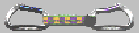# Voronoi Diagrams of 2D Shapes

I have been working on the computation of Voronoi diagrams of planar shapes bounded by straight line segments and circular arcs since 1987. (My own implementation of a divide-and-conquer algorithm for computing Voronoi diagrams formed the basis for my work on NC machining.) During all those years, and while designing and implementing different algorithms, one simple fact became apparent: it is not exactly straightforward to implement a reliable and efficient Voronoi algorithm, but the efforts of implementing a Voronoi algorithm pay off since Voronoi diagrams are very useful for quite a few engineering applications.

May 16, 2000: I have finally managed to start working on WWW pages for vroni, my newest Voronoi algorithm and code. If the theory and application of Voronoi diagrams of points and line segments in 2D is of interest to you then you may want to visit vroni's home page. (The VD code described on this page will be out of date once VRONI is available; please note that I won't hand out that code any longer.)

Related publications:

M. Held (1998):
``Voronoi Diagrams and Offset Curves of Curvilinear Polygons''.
Computer-Aided Design 30(4):287-300, 1998.

The following images show Voronoi diagrams of sample 2D shapes which were computed by means of my Voronoi codes. (Click on an image icon in order to see the full-size image. The full-size images have 1000x700 pixels.)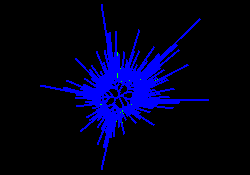This polygonal star consists of 320 contour segments. Computing its Voronoi diagram took 170 milliseconds on a Sun SPARCstation 10. (See also its offset paths, which were computed by means of the Voronoi diagram.)This smooth polygonal shape consists of 8,192 contour segments. Computing its Voronoi diagram took 2,640 milliseconds on a Sun SPARCstation 10. (See also its offset paths, which were computed by means of the Voronoi diagram.)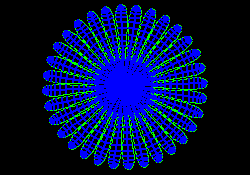This polygonal flower consists of 1,024 contour segments. Computing its Voronoi diagram took 210 milliseconds on a Sun SPARCstation 10. (See also its offset paths, which were computed by means of the Voronoi diagram.)This image shows a zoom into a polygonal spiral which consists of 8,093 contour segments. Computing its Voronoi diagram took 3,140 milliseconds on a Sun SPARCstation 10.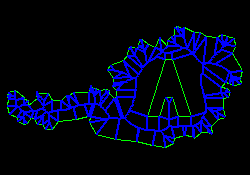This simplified map of Austria contains 1 hole and consists of a total of 116 contour segments. Computing its Voronoi diagram took 80 milliseconds on a Sun SPARCstation 10. (See also its offset paths, which were computed by means of the Voronoi diagram.)The cover logo of my Habilitationsschrift contains 31 curvilinear contours and consists of a total of 189 contour segments (straight lines and circular arcs). Computing its Voronoi diagram took 190 milliseconds on a Sun SPARCstation 10. (See also its offset paths, which were computed by means of the Voronoi diagram.)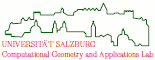file last modified: Friday, 26-Feb-2016 12:34:25 CETCopyright © 2019 Martin Held. All rights reserved.